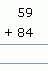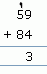Name: ___________________Date:___________________

 Email us to get an instant 20% discount on highly effective K-12 Math & English kwizNET Programs!

Adding double digit - without carryover
Method:
1. Add the numbers in the ones place.
2. Add the numbers in the tens place.
Example:Add the numbers in the ones place = 3+5 = 8
Add the numbers in the tens place = 6+7 = 13Adding double digit - with carryover
Method:
1. Add the numbers in the ones place.
If you get one digit, then place it on the ones place.
If you get two digit number, place the ones digit in the ones place and carry over the tens digit.
2. Add the numbers in the tens place.
Example:

 Question Step 1 Step 2Step 1: Add the numbers in the ones place = 9+4 = 13. Put 3 in the ones place and carry over 1
Step 2: Add the numbers in the tens place with the carry over = 5+8+1 = 14

Directions: Answer the following questions. Also write at least ten examples of your own.Name: ___________________Date:___________________

 Question 1: 57 + 17

 Question 2: 76 + 72

 Question 3: 34 + 47

 Question 4: 87 + 46

 Question 5: 69 + 9

Question 6: This question is available to subscribers only!

Question 7: This question is available to subscribers only!

Question 8: This question is available to subscribers only!

Question 9: This question is available to subscribers only!

Question 10: This question is available to subscribers only!